3-1 嚙踝蕭嚙踝蕭嚙踝蕭嚙踝蕭嚙踝蕭嚙踝蕭嚙踝蕭嚙踝蕭嚙踝蕭嚙踝蕭嚙踝蕭嚙踝蕭嚙踝蕭嚙?

MATLAB 的 plot 指令是最基本的繪圖指令，可以對於一組 x 座標及相對應的 y 座標進行描點作圖，例如：

Example 1: 03-二維平面繪圖/plotxy01.mx = linspace(0, 2*pi); % 在 0 到 2*pi 間，等分取 100 個點 y = sin(x); % 計算 x 的正弦函數值 plot(x, y); % 進行二維平面描點作圖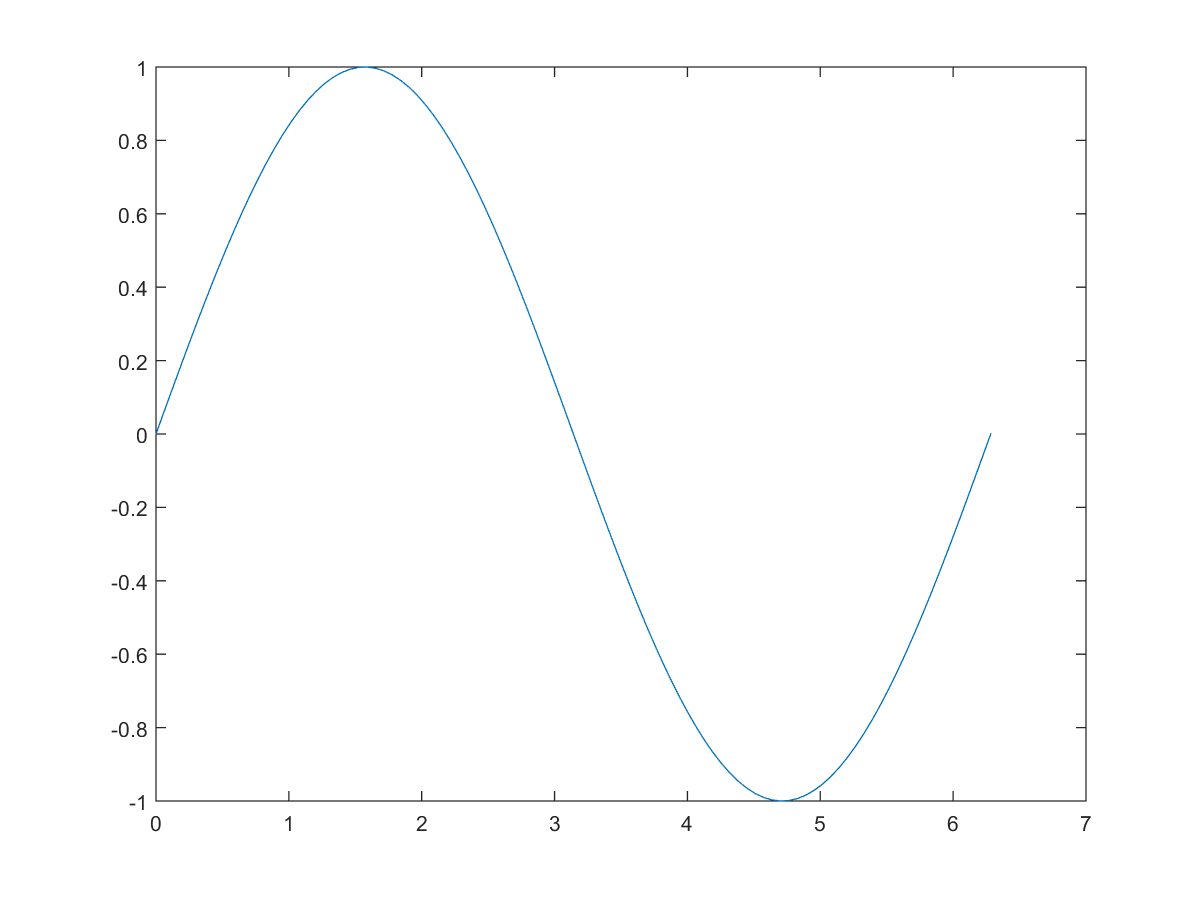Example 2: 03-二維平面繪圖/plotxy02.mx = linspace(0, 2*pi); % 在 0 到 2*pi 間，等分取 100 個點 plot(x, sin(x), x, cos(x), x, sin(x)+cos(x)); % 進行多條曲線描點作圖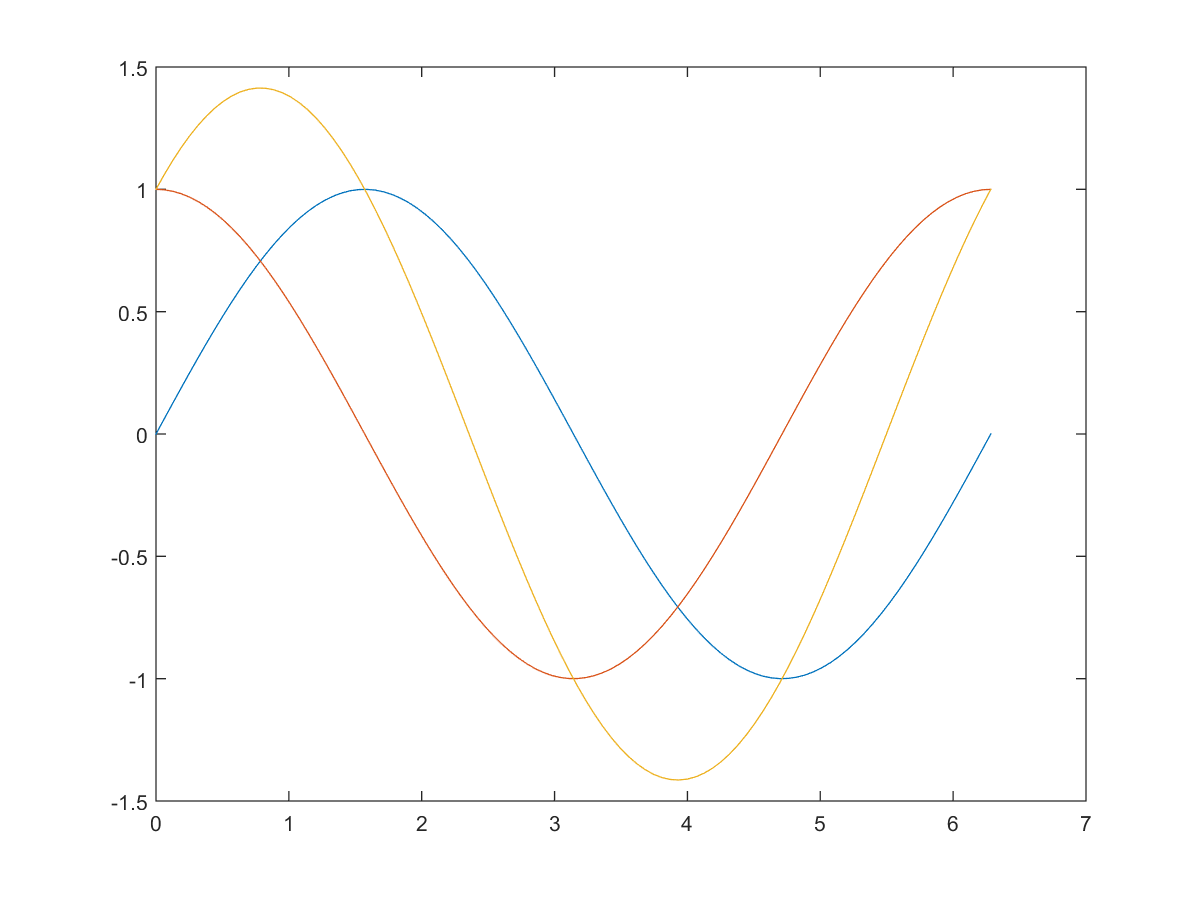MATLAB 在畫出多條曲線時，會自動輪換曲線顏色，以利分辨。（亦可由使用者自行指定曲線顏色及其他相關性質，詳見下一節。）若要以不同的線標（Marker）來作圖，可輸入如下：

Example 3: 03-二維平面繪圖/plotxy03.mx = linspace(0, 2*pi); % 在 0 到 2π 間，等分取 100 個點 plot(x, sin(x), 'o', x, cos(x), 'x', x, sin(x)+cos(x), '*');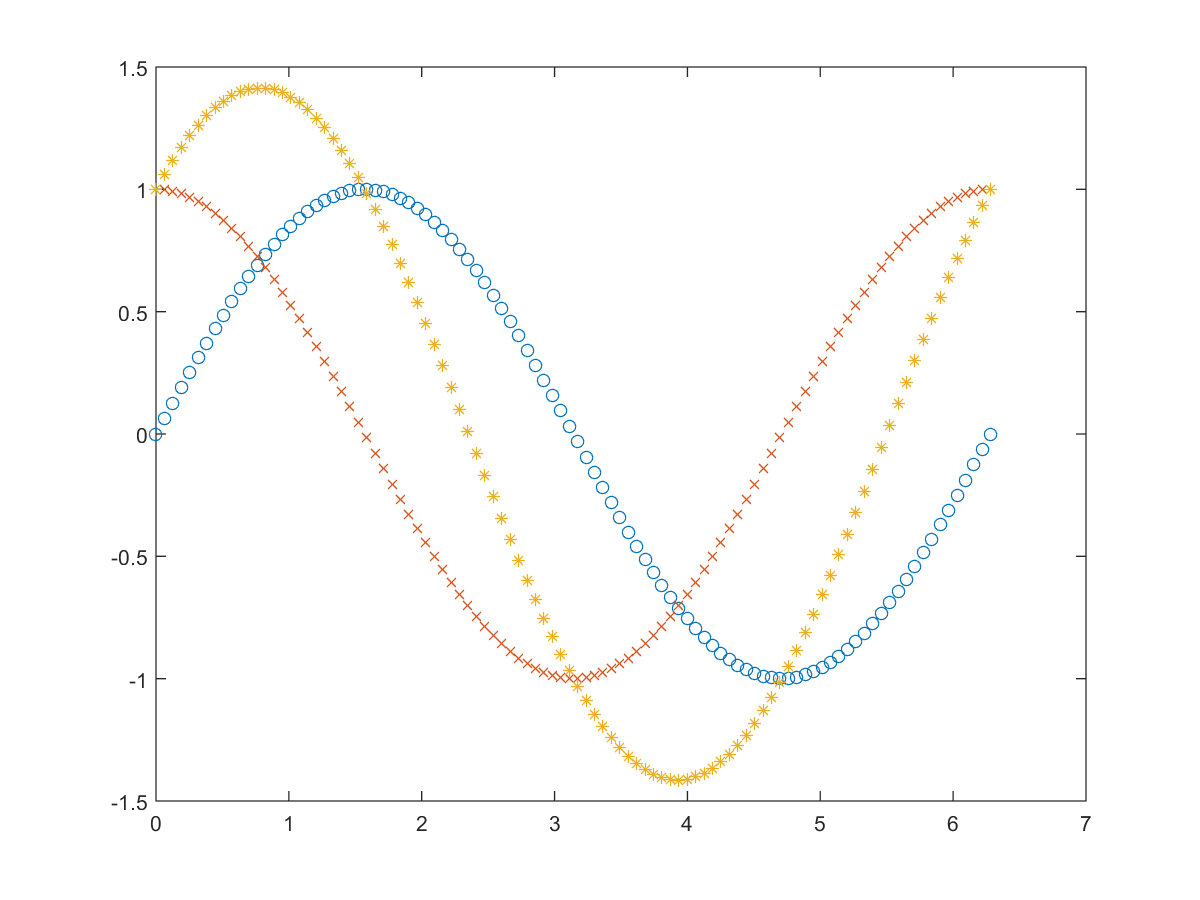Example 4: 03-二維平面繪圖/plotxy04.my = peaks; % 產生一個 49×49 的矩陣 plot(y); % 對矩陣 y 的每一個行向量作圖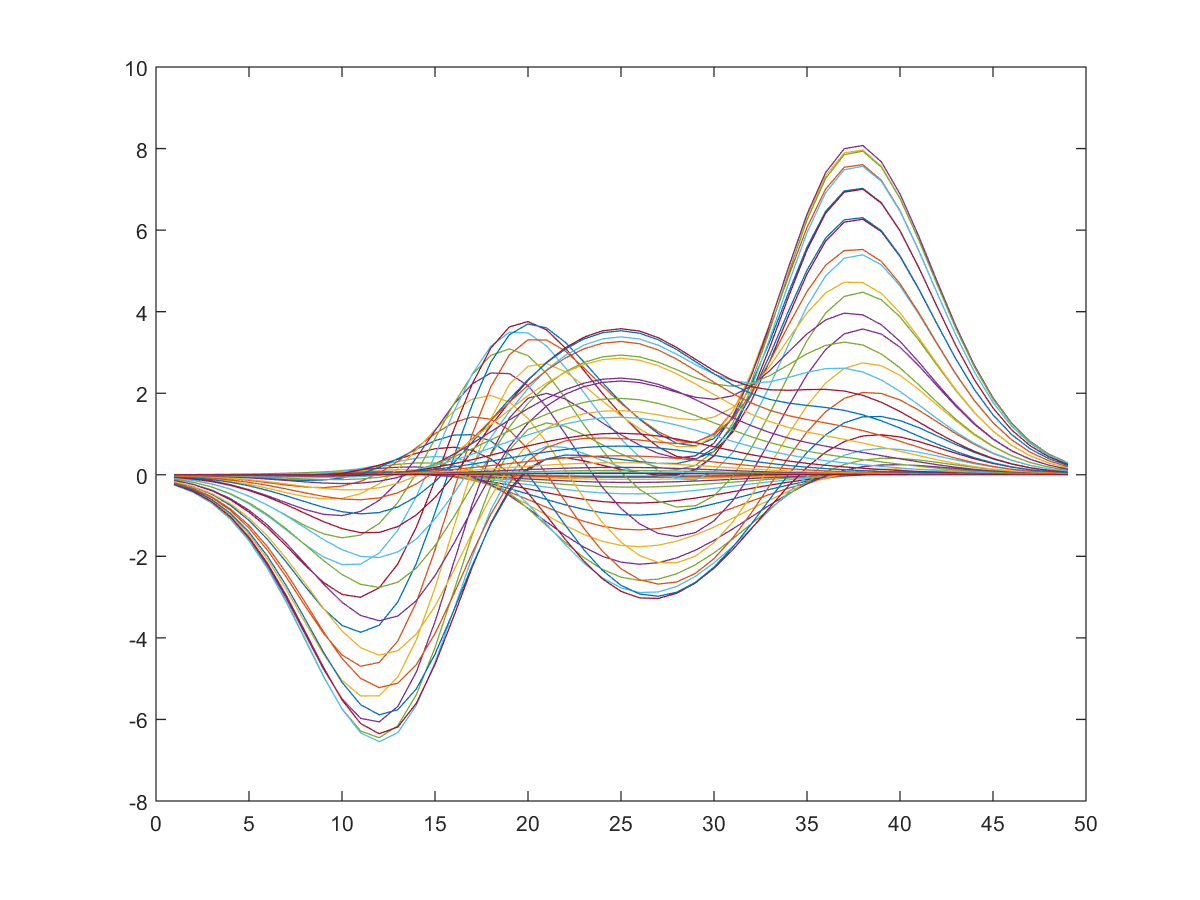Example 5: 03-二維平面繪圖/plotxy05.mx = peaks; y = x'; % 求矩陣 x 的轉置矩陣 x' plot(x, y); % 取用矩陣 x 的每一行向量，與對應矩陣 y 的每一個行向量作圖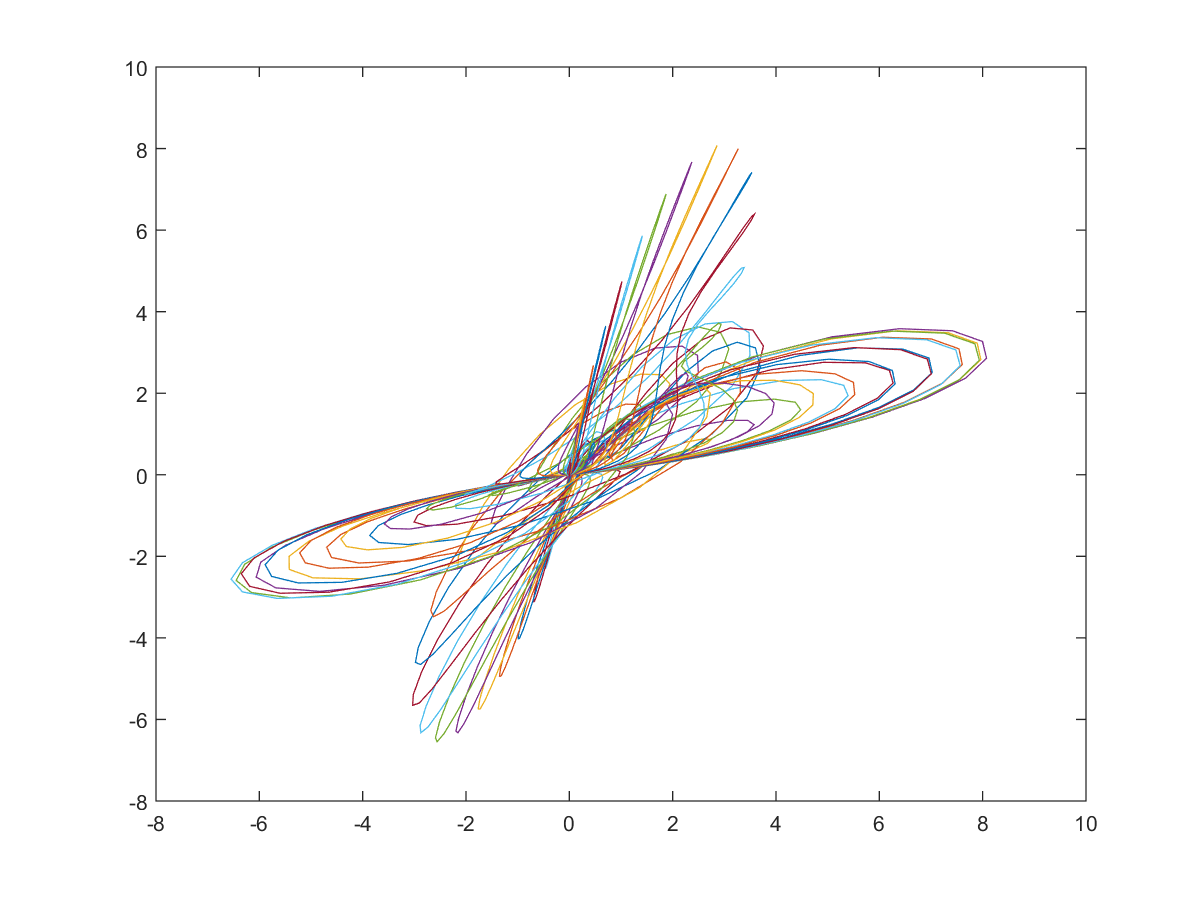Hint

Example 6: 03-二維平面繪圖/plotxy06.mx = randn(30); % 產生 30×30 的亂數（正規分佈）矩陣 z = eig(x); % 計算 x 的「固有值」（或稱「特徵值」） plot(z, 'o') grid on % 畫出格線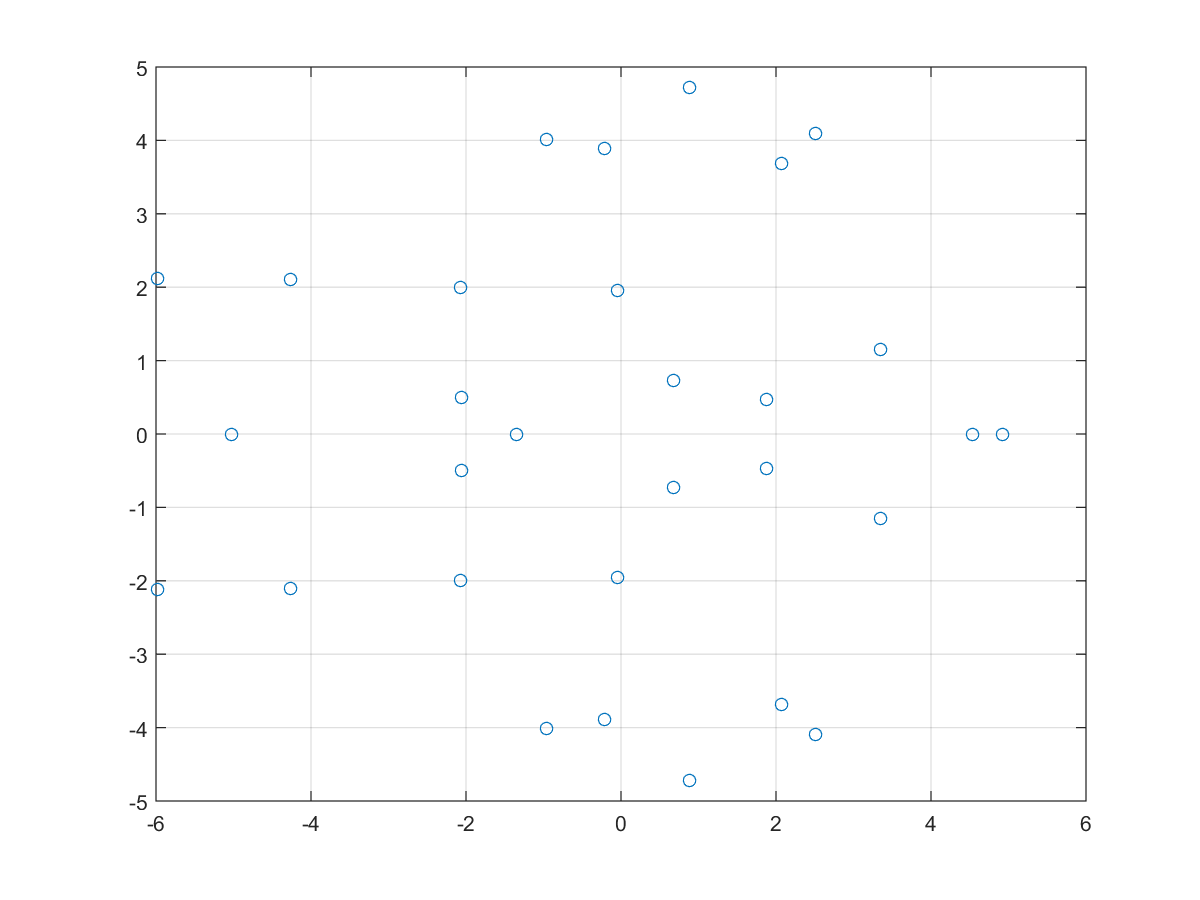Plotx 軸和 y 軸均為線性刻度（Linear Scale）
loglogx 軸和 y 軸均為對數刻度（Logarithmic Scale）
semilogxx 軸為對數刻度，y 軸為線性刻度
semilogyx 軸為線性刻度，y 軸為對數刻度
plotyy畫出兩個刻度不同的y軸

Example 7: 03-二維平面繪圖/plotxy07.mx = linspace(0, 8*pi); % 在 0 到 8π 間，等分取 100 個點 semilogx(x, sin(x)); % 使 x 軸為對數刻度，並對其正弦函數作圖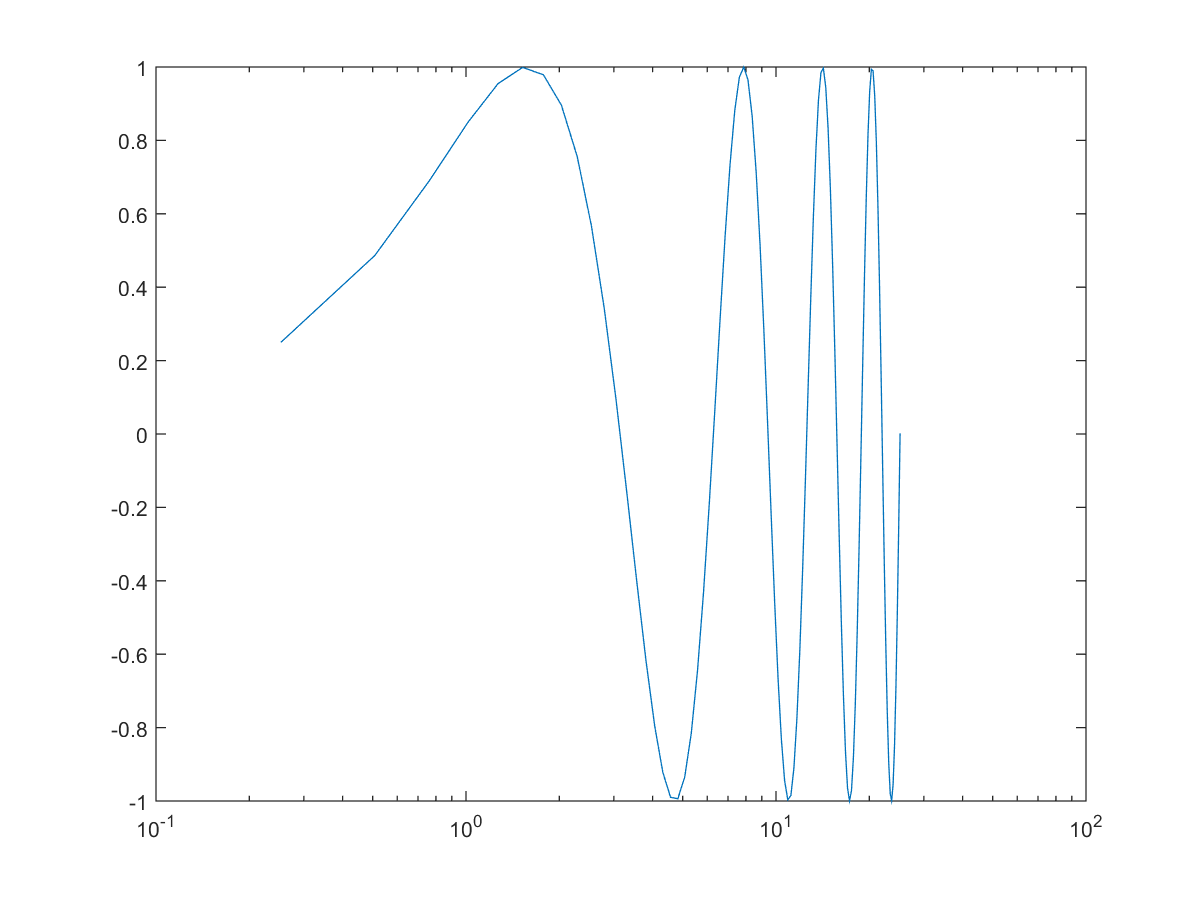Example 8: 03-二維平面繪圖/plotxy08.mx = linspace(0, 2*pi); % 在 0 到 2π 間，等分取 100 個點 y1 = sin(x); y2 = exp(-x); plotyy(x, y1, x, y2); % 畫出兩個刻度不同的 y 軸，分別是 y1, y2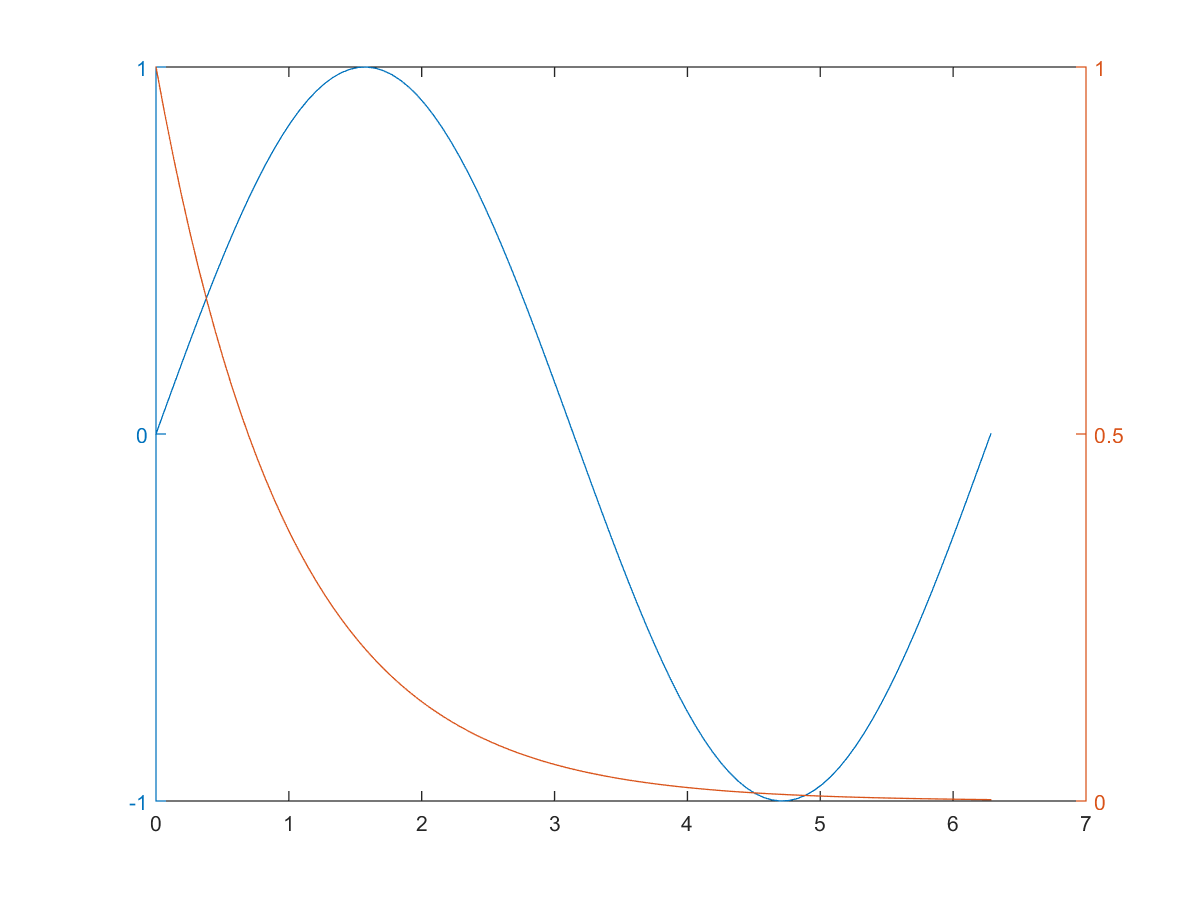MATLAB程式設計：入門篇